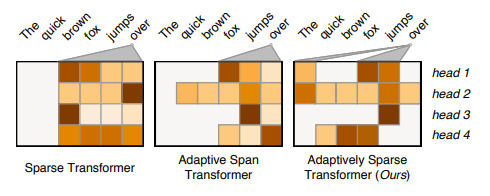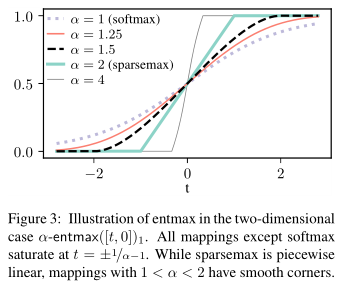By Alexander Rush @srush_nlp

Sparse Sequence-to-Sequence Models¶

Ben Peters, Vlad Niculae, André F.T. Martins

https://arxiv.org/pdf/1905.05702.pdf

Goncalo M. Correia, Vlad Niculae, and Andre F.T. Martins

https://arxiv.org/pdf/1909.00015.pdf

In [ ]:
%%capture
# Code for the paper!
!pip install git+http://github.com/deep-spin/entmax
In [ ]:
#@title Includes

import torch
from torch import nn
import plotly.graph_objects as go
from plotly.subplots import make_subplots
from entmax import sparsemax, entmax15, entmax_bisect

Goals¶

• Learn a transformer model where attention is more sparse in its selection.
• Do so with without hard span constraints on distance.
• Apply an interesting method to a hard task.
• Hopefully discover concrete patterns in the data, and perhaps improve generalization.This note will mostly focus on the technical aspects of how this is done. See paper for full results and details.

Background¶

Key to understanding attention is to really understand softmax used for attention. The standard way of calculation softmax is:

$$\text{softmax}(z)_i = \frac{\exp(z_i)}{\sum_j \exp(z_j)}$$

Softmax is a function that maps from a vector $R^n$ to a probability distribution in the simplex $\Delta^{n-1}$.

Assume we have three possible words we want to attend over A, B, C. and they have scores 1.5, 0, 1. We can visualize how softmax turns these scores in to a probability distribution.

In [ ]:
#@title Simplex Code

rawData = [[0.1, 0.1, 0.8]]
device = "cpu"

def draw_projection(points, mapping="soft", alpha=1):
if mapping == "soft":
raw = torch.softmax(points, dim = -1)
elif mapping == "sparse":
raw = sparsemax(points, dim = -1)
elif mapping == "ent15":
raw = entmax15(points, dim = -1)

#_, argmax = torch.max(points, dim = -1)
#raw = torch.nn.functional.one_hot(argmax)

#raw = torch.softmax(points, dim = -1)
orgData = points.numpy()

rawData = raw.numpy()

fig = make_subplots(rows=1, cols=2, specs=[[{'type': 'scatterternary'}, {'type': 'surface'}]])

'mode': 'markers',
'a': [i for i in map(lambda x: x, rawData)],
'b': [i for i in map(lambda x: x, rawData)],
'c': [i for i in map(lambda x: x, rawData)],
'text': [i for i, _ in enumerate(rawData)],
'marker': {
'symbol': 100,
'color': '#DB7365',
'size': 14,
'line': { 'width': 2 }
}}), row=1, col=1)

for i in range(points.shape):
x= [rawData[i, 1], orgData[i, 1]],
y= [rawData[i, 2], orgData[i, 2]],

z= [rawData[i, 0], orgData[i, 0]],

marker=dict(
size=2
),
line=dict(
width=0.5,
color="blue"

)
),
row = 1, col=2)
x= [0, 0, 1, 0],
y= [0, 1, 0, 0],

z= [1, 0, 0, 1],
marker=dict(
size=4
),
line=dict(
width=5,
color="red"
)
),
row = 1, col=2)

fig.show()
In [ ]:
draw_projection(torch.tensor([[1.5, 0., 1.]]))

For fun here's a bunch of random values. 😄

In [ ]:
randos = torch.rand(100, 3) * 2 - 1
draw_projection(randos)

The trick to reading these "simplex" diagrams is that the corners mean the "more attention" is paid to each of the choices.

Now you might ask, why did we pick that way of mapping from a point to the simplex? It's a good question, and there are many others choices you might make. Here is one called sparsemax:

$$\text{sparsemax}(z) = \arg\min_{\delta \in \Delta} || \delta - z ||$$

This says: simply map to the point in the triangle that is closest in Euclidean distance to the scores. You can show that this will yield sparser solutions, which tend to be on the edges of the simplex. On an edge, one of the choices will be ignored by attention completely. Here's what it looks like. For example here B is not attended to at all.

In [ ]:
draw_projection(torch.tensor([[1.5, 0., 1.]]), mapping="sparse")

Same randoms projected.

In [ ]:
draw_projection(randos, mapping="sparse")

Finally, another really useful thing to know about a distribution is its Entropy denoted as $H$. Entropy represents how uncertain the attention is. Entropy correlates with how far a point on the triangle is from the center.

There are several forms of entropy but the most common for a point $\delta \in \Delta$ is : $$H(\delta) = -\sum_j \delta_j \log \delta_j$$

Here's what it looks like for any attention over 3 values.

In [ ]:
#@title Entropy Code

import plotly.figure_factory as ff
import numpy as np
fig = make_subplots(rows=1, cols=2, specs=[[{'type': 'scatterternary'}, {'type': 'surface'}]])
eps = 1e-5
X = torch.linspace(0, 1.0, 10)
Y = torch.linspace(0, 1.0, 10)
X = X.repeat(10)
Y = Y.repeat(10, 1).t().contiguous().view(-1)
keep = X + Y <= 1.0
X = X[keep]
Y = Y[keep]
Z = 1.0 - X - Y
fig = ff.create_ternary_contour(np.stack([X.numpy(), Y.numpy(), Z.numpy()]) + eps,
entropy.numpy(), interp_mode='cartesian',  showscale=True)
fig.show()

So what's the difference between sparsemax and softmax? Well it seems like starting from the same values, softmax decided on a point that had higher entropy (more red). In fact, you can show that is exactly what is happening. The paper notes an alternative equation for softmax is that it tries to find the closest point while also maximizing entropy.

$$\text{softmax}(z) = \arg\min_{\delta \in \Delta} \delta^{T} z - H(\delta)$$

You can see that as scores get larger the values will move farther from the center, a property known as temperature. Still the values do not end up on the corner.

In [ ]:
v = torch.arange(1, 5)[:, None] * 0.5*  torch.tensor( [[1.5, 0., 1.]])
draw_projection(v)

Model¶

This paper is mainly about exploring variants of these methods in order to induce sparsity into attention of transformer models. The paper first introduces a variant on the ideas above that they will utilize.

The key technique is to introduce Tsallis Entropies $H_\alpha^T$. This generalizes entropy above. The formula is:

$$H_\alpha^T(\delta) = \begin{cases} \frac{1}{\alpha(\alpha-1)} \sum_j (\delta_j - \delta_j^\alpha) & \alpha \neq 1\\ H(\delta) & \alpha= 1 \end{cases}$$

Let's look at this for different alpha values. Notice that they have different pulls.

In [ ]:
#@title Entropy Code
import plotly.figure_factory as ff
import numpy as np
fig = make_subplots(rows=1, cols=2, specs=[[{'type': 'scatterternary'}, {'type': 'surface'}]])
eps = 1e-5
X = torch.linspace(0, 1.0, 10)
Y = torch.linspace(0, 1.0, 10)
X = X.repeat(10)
Y = Y.repeat(10, 1).t().contiguous().view(-1)
keep = X + Y <= 1.0
X = X[keep]
Y = Y[keep]
Z = 1.0 - X - Y
figs = []
for alpha in [0.5, 1.5, 2.0]:
entropy = (1.0 / (alpha * (alpha - 1))) * ((X - X.pow(alpha)) + (Y - (Y+eps).pow(alpha)) + (Z - (Z+eps).pow(alpha)))
fig = ff.create_ternary_contour( np.stack([X.numpy(), Y.numpy(), Z.numpy()]) + eps,
entropy.numpy(), title="alpha %s"%alpha, height = 400,
interp_mode='cartesian',  showscale=True)
figs.append(fig)
fig.show()

So now we can take the formula for softmax above and plug-in a different entropy term. Different values of $\alpha$ correspond to pulling more towards the center (high entropy) or less.

$$\alpha\text{-entmax}(z) = \arg\min_{\delta \in \Delta} \delta^T z - H_\alpha^T(\delta)$$

Furthermore $\alpha = 1$ corresponds to standard softmax, and $\alpha=2$ corresponds to sparse max above.

Let's see what these look like on our attention diagram. Here I will plot the same point on $\alpha = [1, 1.5, 2]$ as the temperature changes. The key things to notices are the smoothness and what happens when you approach a boundary.

In [ ]:
draw_projection(torch.arange(1, 20)[:, None] * 0.1 * torch.tensor([[1.5, 0., 1.]]), mapping="soft")
draw_projection(torch.arange(1, 20)[:, None] * 0.1 * torch.tensor([[1.5, 0., 1.]]), mapping="ent15")
draw_projection(torch.arange(1, 20)[:, None] *0.1 *torch.tensor([[1.5, 0., 1.]]), mapping="sparse")

Here is a similar diagram on their website for the case of attention over two choices.Extensions¶

There are several key extensions in this paper to bring this method beyond this approach above. These include

• Algorithms for computing the $\alpha$-entmax efficiently.
• Computing different $\alpha$ values per head.
• Learning the $\alpha$ adaptively through backpropation.

Experiments¶

This is a paper mostly about getting interpretable results. However the main first aspect is simply demonstrating that they can acheive similar results.

The three models are:

• softmax : standard transformer
• 1.5-entmax: A version of transformer with 1.5-entmax. (This is an "in-between" case where they have a special fast algorithm.)
• $\alpha$-entmax: a transformer with different $\alpha$ per layer and heads.